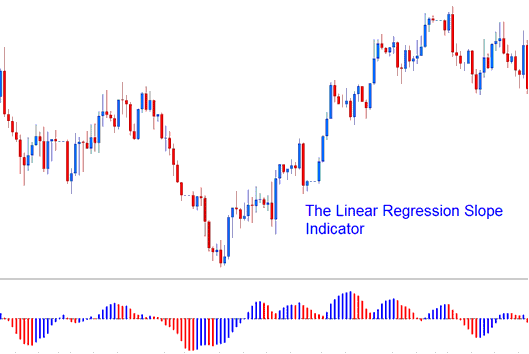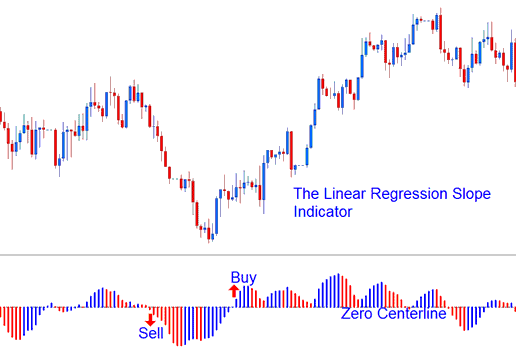# Linear Regression Slope XAUUSD Technical Analysis and Linear Regression Slope XAUUSD Signals

The Linear Regression Slope calculates the slope/gradient value of regression lines which involve the current gold price bar and the previous n-1 gold price bar (where n = regression periods)

This Indicator calculates this value and updates it for each gold price candlestick loaded in the gold price chart.

The Indicator is calculated from the Linear Regression Gold Indicator. The linear regression plots the xauusd trend of the gold price chart over a specified duration of time and this market xauusd trend is determined by plotting a Linear Regression XAUUSD Trend Line using the "least squares fit" method. The slope of this xauusd trend line is then calculated and this forms the linear regression.Linear Regression Slope

The slope values are then smoothed by multiplying the raw slope indicator values by 100 and then dividing this value by the xauusd price

Linear Slope Regression = (raw value of slope * 100 / xauusd price).

The smoothing of the slope values is essential when comparing markets that are volatile and trade within wide gold price ranges for each gold price candlestick. The smoothed slope value will show the percent change in the gold price per every candlestick used to calculate the regression (best fit) line.

## XAUUSD Technical Analysis and Generating XAUUSD Signals

• If the smoothing of the slope is 0.30, then the regression line is rising and adjusting at a rate of 0.30% for every candlestick.

• If the smoothing of the slope of -0.30, then the regression line is going down and adjusting at a rate of -0.30% for every candlestick.

The regression slope is displayed as a bi-color histogram that oscillates above and below zero center line. The center line that is used to generate xauusd trading signals is set at the 0 level.

• A rising slope (greater than the previous value of 1 candlestick ago) is displayed in the Blue/Upward Slope color,

• A declining slope (lower than the previous value of 1 candlestick ago) is displayed in the Red/Downward Slope color.Technical Analysis